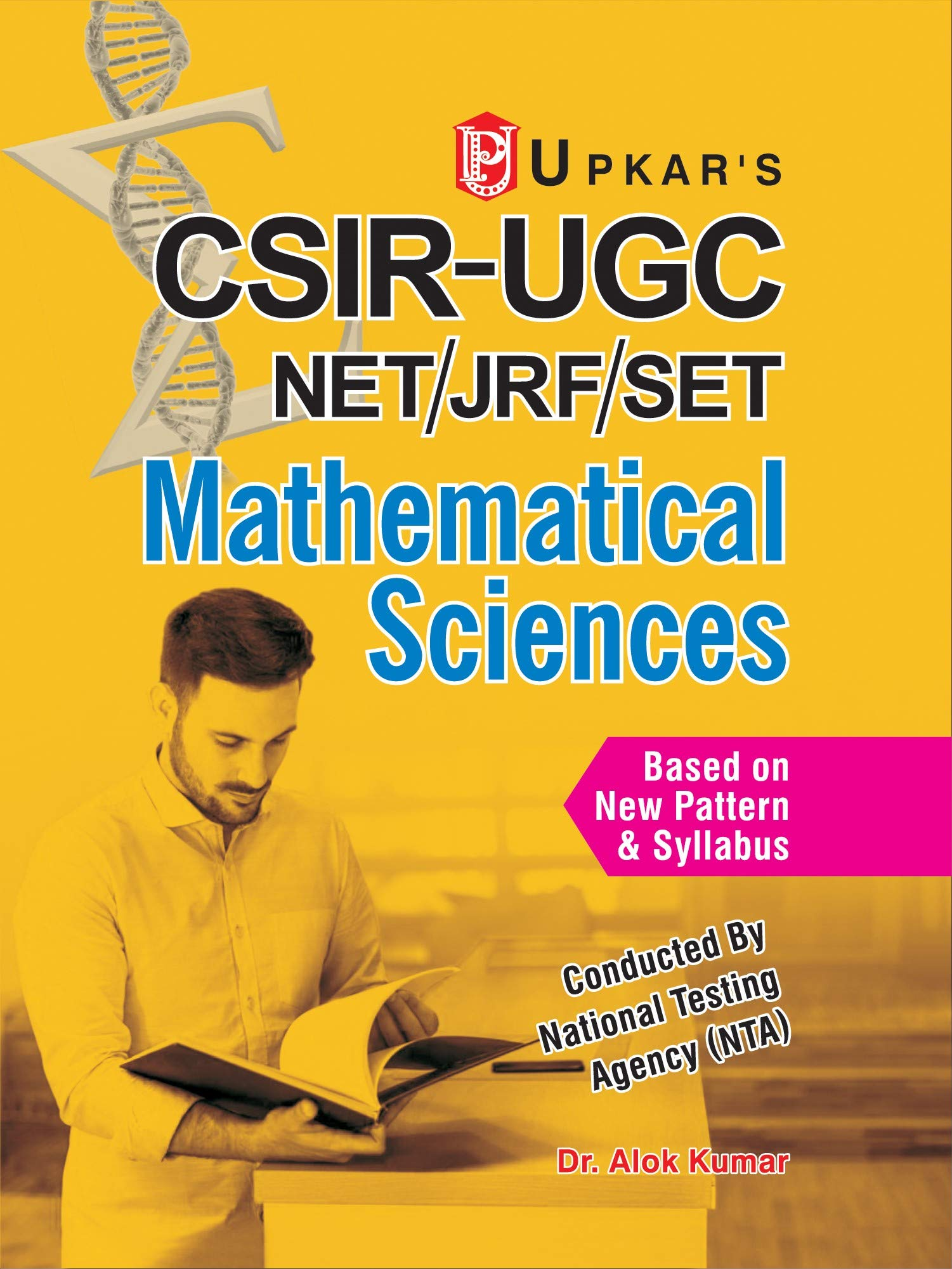# Upkar’s CSIR-UGC Net/JRF/SET Mathematical SciencesPrice: ₹539.00
(as of Jun 08,2023 12:30:13 UTC – Details)

Table of contents paper-I section (a) computer awareness and scientific aptitude computer awareness scientific aptitude section (b) 1. Basic concepts of real and complex analysis 2. Basic concepts of linear algebra 3. Basic concepts of probability 4. Linear programming basic concepts 5. Real analysis 6. Complex analysis 7. Algebra 8. Linear algebra 9. Differential equations 10. Operational research modelling 11. Linear programming paper-ii 1. Real analysis 2. Complex analysis 3. Algebra & advanced algebra 4. Advanced analysis 5. Functional analysis 6. Topology 7. Discrete mathematics 8. Ordinary and partial differential equations 9. Number theory 10. Mechanics 11. Fluid mechanics 12. Differential geometry 13. Calculus of variation 14. Linear integral equations 15. Numerical analysis 16. Integral transform 17. Mathematical programming 18. Measure theory top

Publisher‏:‎Upkar Prakashan; 2017th edition (1 December 2008)
Language‏:‎English
Paperback‏:‎976 pages
ISBN-10‏:‎8174823808
ISBN-13‏:‎978-8174823809
Item Weight‏:‎200 g
Dimensions‏:‎20 x 14 x 4 cm